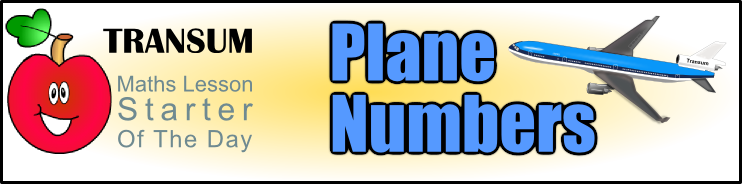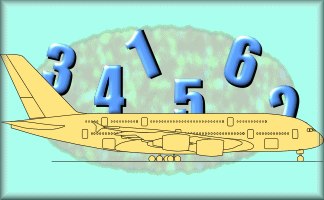###Arrange the numbers on the yellow tiles so that the sum of the numbers in the vertical line is the same as the sum of the numbers in the horizontal line

1

2

3

4

5

6

How many different ways are there of doing this task?

A self-checking, interactive version of this activity with 30 different puzzles is here.

This is the
projectable version
of this activity. See
interactive version
designed for
pupils to use.

Topics: Starter | Arithmetic | Number | Problem Solving

• K Hudson, Drigg
•
• Looks easy but proved quite tricky. Nice one!
• Helen McKenna, Elgin
•
• Hard to start, but then easy. We found 10 different solutions in 5 minutes.
• Class 2, Blackboys Primary School
•
• Ethan Peck found it a tough challenge, but soon got the hang of it. "It was really fun but tricky," says Poppy. "I enjoyed the challenge and found 1 solution," commented Kizzifer Budd. Top challenge.
• Mr M's Year 7s, CCS, Bali
•
• We noticed that you can only have an odd number in the middle (ie. 1,3 or 5). The total number is either 11,12 or 13 depending on what number you put in the middle. Great starter, thanks. :D.
•
• Enjoyed but kinda difficult!
• Paula, Coln House
•
• I teach in a SEBD secondary school, these starters help the classes to settle and they (the pupils) (mostly) enjoy the challenge. Sometimes we might spend longer on a problem if the pupils are very engaged.
If we vote on a starter I always ask for their input and comments.
For more practical starters we often cut up paper and model the problem, I find this helps , I also try to create a sense of controlled urgency as this helps the pupils concentrate and focus.
Sometimes the starters are too complex, or wordy, so I quickly search and find a more appropriate problem.
I think these starters are very useful as you can extract lots of mathematics from them to suit your particular level, style and type of class.
Thank you.
• Mrs Clay's Y5 Maths Set GMS, Bedfordshire
•
• We found three possible solutions, because we would not allow jiggling of the digits eg we think 4+1+6= 2+1+3+5 is the same as 6+1+4= 3+1+2+5 and the digit used twice must be odd. :)
4+1+6 = 2+1+3+5
5+3+4 = 6+3+2+1
6+5+2 = 4+5+1+3.
• Dan's Dad, Milton Keynes, UK
•
• There's a reason why the number in the middle must be odd:
First add up all the numbers: 1+2+3+4+5+6=21
If you put an odd number in the middle, for example 1, the numbers that are left now add up to 20.
And 20 can be split into two equal halves of 10
i.e. 6+4 and 2+3+5 both equal 10
6+1+4 = 2+1+3+5
Using any odd number in the middle will leave you with equal halves to share up and across
If you try it with a 2 in the middle, you are left with the numbers#
1+ 3+4+5+6=19
And 19 cannot be shared equally up and across.
• Mr Eagle, Eagletown
•
• My mixed year 5/6 thought it was an absolute doddle. They thoroughly enjoyed the compexities of this challenge and look forward to the next one!
• Mr. Townsend, Harmondsworth Primary Y3/4
•
• We found one solution as a starter activity. We used 4, 3, 5 in the vertical column and 1,3,2,6 in the horizontal. It was challenging!
• TKe, Hull
•
• After finding how many 'unique' solutions, get the class to calculate how many permutations there are.
•
• We found these a little tricky but if we had more time, we would have found more.
• Mr G, Kenya
•
• My class loved this. We found 3 solutions in total not including moving the numbers to different locations within their row or column - addition is commutative so it didn't matter where the numbers location was.
There were 3 solutions and you hade to have an odd number in the central square.

How did you use this starter? Can you suggest how teachers could present or develop this resource? Do you have any comments? It is always useful to receive feedback and helps make this free resource even more useful for Maths teachers anywhere in the world.

Previous Day | This starter is for 3 February | Next Day

Your access to the majority of the Transum resources continues to be free but you can help support the continued growth of the website by doing your Amazon shopping using the links on this page. Below is an Amazon link. As an Amazon Associate I earn a small amount from qualifying purchases which helps pay for the upkeep of this website.

Educational Technology on AmazonTeacher, do your students have access to computers such as tablets, iPads or Laptops?  This page was really designed for projection on a whiteboard but if you really want the students to have access to it here is a concise URL for a version of this page without the comments: Transum.org/go/?Start=February3 However it would be better to assign one of the student interactive activities below.Here is the URL which will take them to the interactive version of this activity with 30 different variations.

Transum.org/go/?Num=6For All: